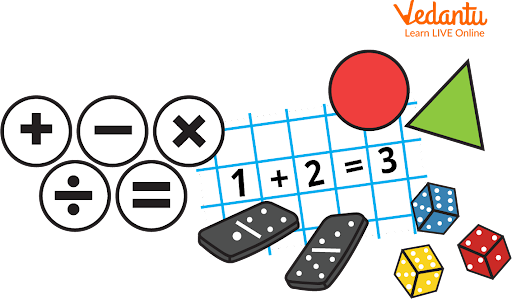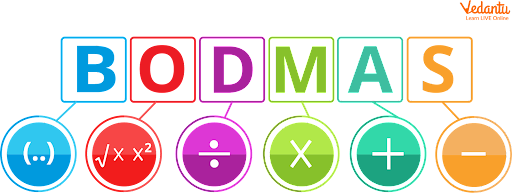Courses
Courses for Kids
Free study material
Free LIVE classes
More

# BODMAS Rule: BODMAS Questions and Answers## Introduction to BODMAS

Math is all about logic and following a few simple BODMAS rules to make our calculations go more smoothly. BODMAS was invented by Achilles Reselfelt, a mathematician. It's a mnemonic for remembering how to evaluate mathematical operators in a mathematical statement with several mathematical operations. It is the simplest way to solve an expression with multiple operators.

BODMAS is used to simplify an expression or equation which is combined two or more numbers with the most often used mathematical operators: Addition (+), Subtraction (-), multiplication (x) and Division (÷).Mathematical Operators

## What is BODMAS?

The order of operations, or BODMAS, is a sequence for performing operations in an arithmetic statement. As a result, BODMAS is one of the basic principles for simplifying multiple-operator expressions. The BODMAS rule does not cover equations. It can be used on mathematical expressions with many operators.

## BODMAS Rule

Have you ever thought about how long mathematical expressions having more than two operators are simplified? According to the BODMAS rule, mathematical expressions with numerous operators must be solved from left to right in the BODMAS order. Division and multiplication are interchangeable and are determined by which comes first in the phrase, as are addition and subtraction.

### BODMAS Stands for:-

B - Bracket

O - Order of (square, indices etc.)

D - Division

M - Multiplication

S - SubtractionBODMAS Rule

## How to Apply BODMAS?

When there are several operators in an expression, the BODMAS rule can be used. In that instance, we first simplify the brackets from the innermost to the outermost [()], then assess the values of exponents or roots, simplify multiplication and division, and finally perform addition and subtraction operations while moving from left to right.

Let’s start simplifying expressions in the following order

1. Bracket: Calculate everything inside the bracket first.

For example:

$4 \times(12-9)$

$=4 \times 3$

$=12$

The correct answer is 12 by using the BODMAS rule.

1. Order of: Solve the power, square etc.

For example:

$5+4^{2}$

$=5+16$

$=21$

The correct answer is 21 by using the BODMAS rule.

1. Division and Multiplication: Since multiplication and division are equally important, they should be completed from left to right.

For example:

$6+24 \div 3 \times 2$

$=6+8 \times 2$

$=6+16$

$=22$

The correct answer is 22 by using the BODMAS rule.

1. Addition and Subtraction: Since addition and subtraction are equally important, they should be completed from left to right.

$21+(8-5)$

$=21+3$

$=24$

The correct answer is 24 by using the BODMAS rule.

## BODMAS without Brackets

We can also apply this rule if there are no brackets; go to indices, then multiplication and division, and finally addition and subtraction. One instructs you to multiply first, then divide, whereas the other instructs you to do the opposite.

### Here is a BODMAS Example with Answer.

Q. Simplify $3+4 \times 2+4-1$

Ans. BODMAS says Multiplication first,

so multiply, $4 \times 2$

$3+8+4-1$

$3+8+4=15$

Now perform subtraction at last

$15-1=14$

The correct answer is 14 by using the BODMAS rule.

## BODMAS Rule Problems

Problem 1: Simplify $12 \div 4 \times 2+3^{2}-(9+4)$.

Solution: Using the BODMAS Rule (left to right whichever operations come first we will follow that).

$12 \div 4 \times 2+3^{2}-(9+4)$

First we will simplify bracket,

$=12 \div 4 \times 2+3^{2}-13$

Now we will simplify powers,

$=12 \div 4 \times 2+9-13$

Now we will divide 12 by 4,

$=3 \times 2+9-13$

Now we will multiply 3 and 2,

$=6+9-13$

Now we will add and subtract,

$=15-13$

$=2$

Problem 2: Simplify the expression by using the BODMAS rule: $(9 \times 3 \div 9+1) \times 3$.

Solution: Step 1: Using BODMAS Rule (left to right whichever operations come first we will follow that). Here, first, we simplify the bracket and inside the bracket, we will multiply first then division (we can do vice versa) and then addition. Thus, we need to multiply 9 by 3 in the given expression,

$(9 \times 3 \div 9+1) \times 3$ and we get,

$(27 \div 9+1) \times 3$

Step 2: Now, we need to divide 27 by 9 inside the bracket, and we get, $(3+1) \times 3$

Step 3: Remove the parentheses after adding 3 and 1 ,

we get, $4 \times 3$

Step 4: Multiply 4 by 3 to get the final answer, which is 12.

$\therefore(9 \times 3 \div 9+1) \times 3=12$

## Summary

The BODMAS is a sequence for performing operations in an arithmetic statement. Here B stands for Brackets, O for Order of powers or roots, D for Division, M for Multiplication A for Addition, and S for Subtraction. This indicates that expressions with numerous operators must be simplified in this order only, from left to right. We start with brackets, then powers or roots, division or multiplication (whichever comes first from the left side of the expression), and finally subtraction or addition (whichever comes first from the left side of the expression).

If there are no brackets, we utilise the BODMAS rule to get the desired result. If there are no brackets, start with 'order' or 'of,' then divide or multiply (whichever comes first from left to right), and last add or subtract (whatever comes first from left to right).

Q1. Simplify the expression by using the BODMAS rule: $3+4 \times 2+4-1$.

Ans: 14

Q2. Simplify the expression by using the BODMAS rule: $(27 \div 9+1) \times 3$.

Ans: 12

Last updated date: 26th Sep 2023
Total views: 125.1k
Views today: 1.25k

## FAQs on BODMAS Rule: BODMAS Questions and Answers

1. Do you use BODMAS when working with fractions?

BODMAS and BOMDAS do not address powers (also known as exponents or indices), fractions, and roots, but we still need to know how to manage them. Fractions, powers, roots, and other self-contained elements of expressions should be handled as if they were in brackets, i.e. they should be worked out first. We still need to know how to deal with powers (also known as exponents or indices), fractions, and roots even though they are not addressed by BODMAS. Work out any fractions, powers, roots, and other self-contained expression components first as if they were in brackets.

2. In an equation, what is the order of operations?

A rule that specifies the right procedure for evaluating a mathematical equation is known as the order of operations. PEMDAS - Parentheses, exponents, multiplication and division (from left to right), addition and subtraction are the steps that we can remember in that order using PEMDAS (from left to right).

The full form of BODMAS is – Brackets Order Division Multiplication Addition and Subtraction. PEMDAS is used mainly in the US but in India and the UK, we call it BODMAS. There is no difference between them.

3. When should you use BODMAS?

When completing a mathematical problem including many operations, BODMAS assists you in determining the order to execute them. Anything in brackets should be completed first, followed by the ordering, any division or multiplication, and lastly addition or subtraction.

Thus, BODMAS enables you to choose which order to carry out the various operations in a mathematical number phrase including numerous separate operations.